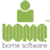▲ 0 ▼ ♥ 0 Can BOME Midi Translator remap/rearrange launchpad notes?  i.e., instead of each subsequent pad being a semitone up (chromatic), I’d like to have it be say “Lydian” scale.  After playing around with the software, the only way I could fathom doing this is the following: Add translator Name translator (for example) “C#2 to Launchpad Pad 1” Set incoming to “midi message,” “note on,” Under  “note” drop down select the note being triggered by looking at the monitor and “capture midi” (don’t change velocity scaling.  Leave exactly as how launchpad pro picks up) Set outgoing to desired note depending on “Lydian” scale Repeat for each of the 64 pads of the launchpad pro….meaning there will be 64 translators to make.  You can imagine how cumbersome this would be Is there a way to accomplish this via scripting?  Say, a script that takes in a series of notes (for any desired scale) and laying it out sequentially on pads of the launchpad. I know the Launchpad Pro Mk2 now has open-source firmware that allows you to go in a program this.  But I want to be able to apply this to other midi controllers that aren’t open source (DJ techtools midi fighter 64, Ableton Push 2, Maschine, Akai MPC, etc). A more general question.  Is it possible to make only one translator with a giant script that accomplishes the above? Marked as spamPosted by Oliver Oliverio (Forum: 4, Answers: 1) June 2, 2020 7:45 am 40 views
 ▲ 0 ▼ ✔ Private answer Hi, Yes this can be done. If I know the exact formula I would provide you that but I don't so another way would be where you look at the incoming note pp and set the outgoing note qq.   if pp==0 then qq=0 if pp==1 then qq=2 if pp==2 then qq=4 if pp==3 then qq=6 if pp==4 then qq=7 if pp==5 then qq=9 if pp==6 then qq=11 if pp==7 then qq=12 if pp==8 then qq=14 ... and so forth Use pp as incoming note and qq as outgoing note. This is based on C Lydian. If you want to use a different scale you could use an off set at the beginnint to adjust the incoming note to the scale key that you want.   Steve CaldwellBome Q and A Moderator andIndependent Bome Consultant/Specialistbome@sniz.biz Marked as spamPosted by Steve-Bome Forum Moderator (Forum: 50, Answers: 5308) June 2, 2020 3:50 pm ▲ 0 ▼ ✔ Private answer Thx Steve, just for clarity.  Do those numbers above correspond to absolute note numbers in standard midi? (1-128) Where middle C is note 60, while 21 (A1...or is it A0?) and 108 (C8)are the lowest/highest notes on an 88 key piano.   As a test case, say I want to map the first three white keys starting from middle C (note 60) a third above (transposed a 3rd).  Let me know if this is how you'd write this translator.     Translator name - "Transpose a 3rd up" Incoming -> midi message -> note on select channel corresponding to midi keyboard set variable to note "pp" in the rules section   // if note is C, play E if pp = 60 then qq = 64   // if note is D, play F if pp = 62 then qq = 65   // if note is E, play G if pp = 64 then qq = 67 set outgoing to midi message, note on.   Here's where I'm stuck, where do I specify the variable qq as midi note output?   This is more for those who are familiar with scripting/programming, but will there be a feature that wrap all of the above into an IDE?  (Rather than click through drop down menus and making selections, if we could do that with variable assignments, if/else statements, and for/while loops that would be awesome.     Marked as spamPosted by Oliver Oliverio (Forum: 4, Answers: 1) June 3, 2020 6:45 am ▲ 0 ▼ ✔ Private answer Hi Oliver, For outgoing action, just put qq in the box for the out outgoing note number on the output side over on the right. See attachment. As far as loops, the way to do it with MT Pro is repeating timers where the timer increments or decrements a global variable each time it is called. I also took the liberty of creating a translator that will convert from a Chromatic to Lydian Scale using math. The way it works is we define the note conversion in a single octave and then we expand to  use with any octave I set it up so you can use any starting note. It the attached case I used note 60. Here are the rules. The input note is variable pp and the output note is variable qq. //offset if any // 0 = c , 1=c# 2=d etc starting at c-2 // This is the starting low note that you // want to start your Lydian Scale // The upper note will be adjusted automatically // but never greater than MIDI 127 ga=60 vv=pp-ga if vv<0 then exit rules, skip Outgoing Action // Get Mod for 7 notes , interval is in tt rr=vv%7 if rr==0 then tt=rr if rr==1 then tt=rr+1 if rr==2 then tt=rr+2 if rr==3 then tt=rr+3 if rr==4 then tt=rr+3 if rr==5 then tt=rr+4 if rr==6 then tt=rr+5 // tt is now the interval for the incoming note // Octave adjust ss=vv/7 uu=ss*12 tt=tt+uu tt=tt+ga // don't go beyond MIDI limits if tt<0 then exit rules, skip Outgoing Action if tt>127 then exit rules, skip Outgoing Action I hope I got the math right. This methodology should be able to be applied to any scale you wish.     Steve Caldwell Bome Q and A Moderator and Independent Bome Consultant/Specialist bome@sniz.biz Marked as spamPosted by Steve-Bome Forum Moderator (Forum: 50, Answers: 5308) June 3, 2020 2:28 pm